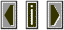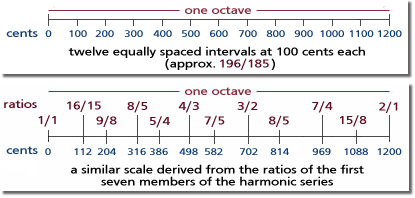CentsThe measurement of interval size by the use of a scale which divides the octave into 1200 equal divisions known as cents.

 The equal tempered tuning system in common use divides the octave into 12 equal divisions, each of which is 100 cents in size. The cent system of interval measurement is useful for the analysis and specification of microtonal scales.